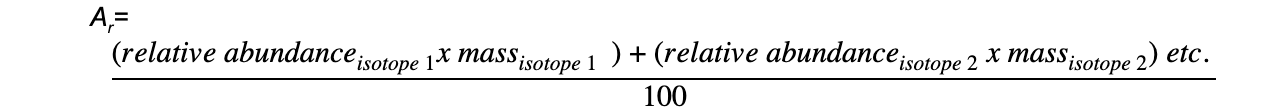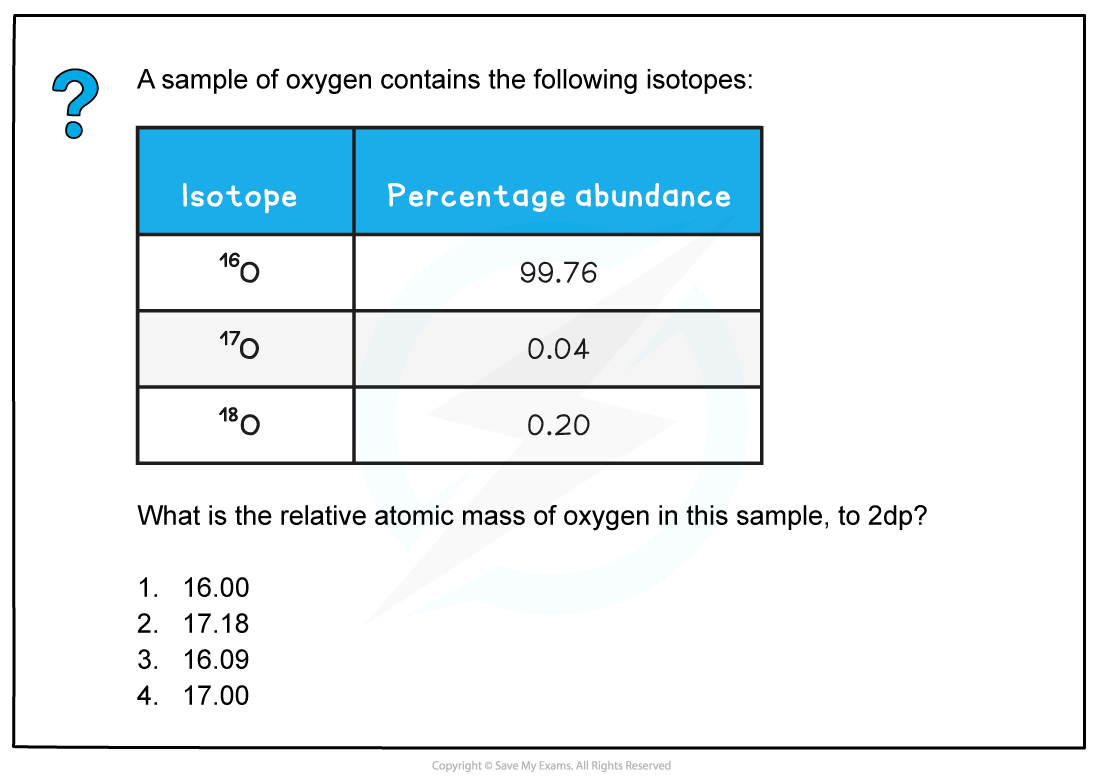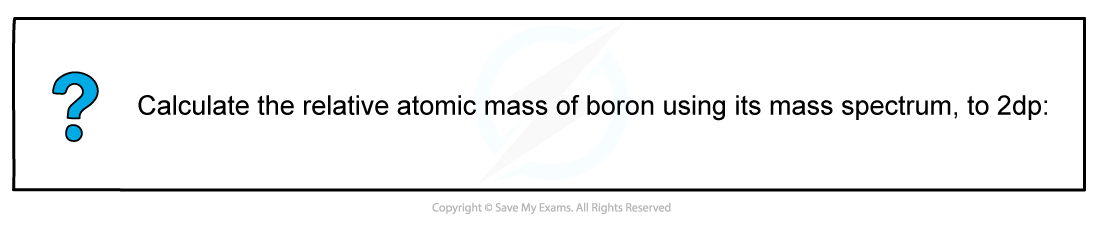# CIE A Level Chemistry复习笔记4.1.3 Isotopic Abundance & Relative Atomic Mass

### Calculating Relative Atomic Mass

• Isotopes are different atoms of the same element that contain the same number of protons and electrons but a different number of neutrons.
• These are atoms of the same elements but with different mass numbers
• Because of this, the mass of an element is given as relative atomic mass (Ar) by using the average mass of the isotopes
• The relative atomic mass of an element can be calculated by using the relative abundance values
• The relative abundance of an isotope is either given or can be read off the mass spectrum#### Worked example: Calculating relative atomic mass of oxygenThe correct answer is 1= 16.0044

= 16.00

#### Worked example: Calculating relative atomic mass of boron